# 不到1美元！1小时内训练自己的中文词向量 – Amazon SageMaker BlazingText nothing is impossible

SageMaker是AWS上一个托管的机器学习平台服务，能够帮助客户高效的自动标注数据、训练和部署机器学习模型。SageMaker内置了二十多种AWS优化过的机器学习高性能算法，包括常见的线性回归、神经网络、时间序列等，并且还支持MXNet、TensorFlow、PyTorch等主流深度学习框架。今天我们将介绍如何使用Amazon SageMaker服务的BlazingText在一小时内快速训练wiki中文的词向量。

Amazon SageMaker BlazingText 算法提供了 Word2vec 和文本分类算法的高度优化的实现。利用 BlazingText 算法，您可以轻松扩展到大型语言数据集。与 Word2vec 类似，它提供了 Skip-gram 和持续单词袋 (CBOW) 两种训练架构来训练词向量，称之为（WordEmbedding），并且还实现了监督学习的fastText文本分类器，您可以使用多台CPU服务器器 或 GPU 服务器在几分钟内对超过 10 亿个单词的模型进行训练。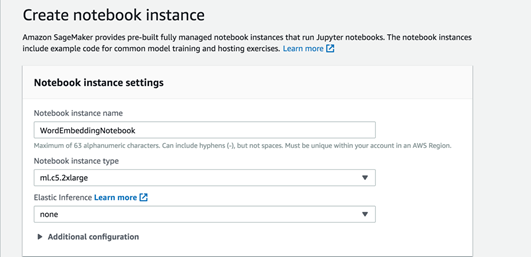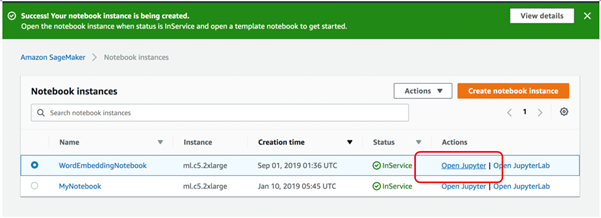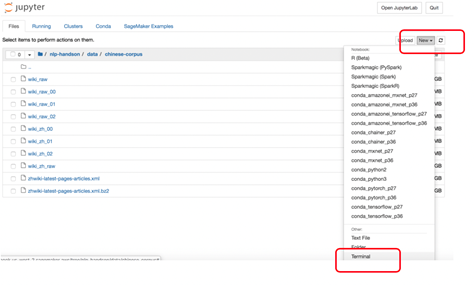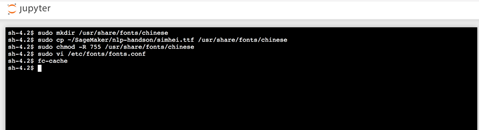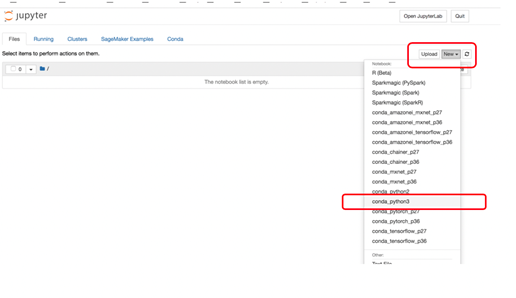``````importsagemaker

fromsagemaker import get_execution_role

importboto3

importjson

sess = sagemaker.Session()

role = get_execution_role()

print(role) # This is the role that SageMaker would use to leverage AWS resources (S3, CloudWatch) on your behalf

bucket = '421710401846-sagemaker-us-west-2'# Replace with your own bucket name if needed

prefix = 'nlp-handson'#Replace with the prefix under which you want to store the data if needed ``````

``!wget https://421710401846-sagemaker-us-west-2.s3-us-west-2.amazonaws.com/nlp-handson/zh-train/wiki_zh_full  ``

• 原始数据包含许多英文单词，阿拉伯数字和标点符号，删除这些噪音信息
• 使用 Jieba分词工具解析中文单词，以空格分开。因为在解析单词时，我们会遇到很多毫无意义的中文词，如 “的、有然、因为”，我们称这些单词停止词（Stopwords），也需要删除
• ``````# Install jieba tool

!pip install jieba

!wget https://421710401846-sagemaker-us-west-2.s3-us-west-2.amazonaws.com/nlp-handson/zhstopwords.txt

importlogging,jieba,os,re

defget_stopwords():

basicConfig(format='%(asctime)s:%(levelname)s:%(message)s',level=logging.INFO)

#加载停用词表

stopword_set = set()

with open("zhstopwords.txt",'r',encoding="utf-8") as stopwords:

for stopword in stopwords:

return stopword_set``````

``````defparse_zh_words(read_file_path,save_file_path):

#过滤掉英文和数字等特殊字符

r1 = '[^\u4e00-\u9fa5]'

#写文件

output = open(save_file_path,"w+",encoding="utf-8")

#获取停用词表

stopwords = get_stopwords()

#定义一个字符串变量，表示一篇文章的分词结果

article_contents = ""

while content_line:

content_line = content_line.strip("\n")

if len(content_line) > 0:

#去除数字和英文等特殊字符

zh_content_line = re.sub(r1, '', content_line)

#使用jieba进行分词

words = jieba.cut(zh_content_line,cut_all=False)

……

input_file = './data/wiki_zh_full'

output_file = './data/wiki_zh_corpus'

parse_zh_words(input_file, output_file)  ``````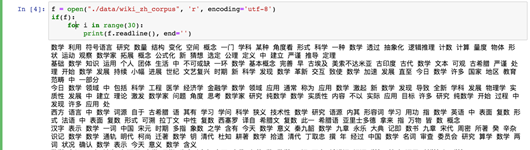``````train_channel = prefix + '/zh-train'

s3_train_data = 's3://{}/{}'.format(bucket, train_channel)

s3_output_location = 's3://{}/{}/zh-output'.format(bucket, prefix)  ``````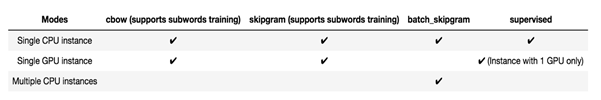``````container = sagemaker.amazon.amazon_estimator.get_image_uri(region_name, "blazingtext", "latest")

bt_model = sagemaker.estimator.Estimator(container,

role,

train_instance_count=1,

train_instance_type='ml.p3.2xlarge',

train_use_spot_instances = True,

train_volume_size = 10,

train_max_run = 3600,

train_max_wait = 3600,

input_mode= 'File',

output_path=s3_output_location,

sagemaker_session=sess)  ``````

``````set_hyperparameters(mode= "skipgram", #"batch_skipgram",

epochs=10,
min_count=5,
sampling_threshold=0.0001,
learning_rate=0.05,
window_size=5,
vector_dim=300,
negative_samples=5,
batch_size=11,
evaluation=False
subwords=False)

train_data = sagemaker.session.s3_input(s3_train_data, distribution='FullyReplicated', content_type='text/plain', s3_data_type='S3Prefix')
data_channels = {'train': train_data}
``````

``fit(inputs=data_channels, logs=True) ``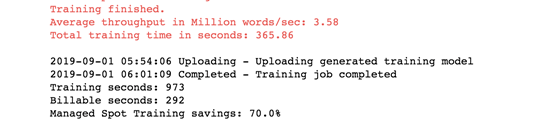``````frommanifold importTSNE

frommatplotlib import pylab

frompylab import mpl

tsne = TSNE(perplexity=40, n_components=2, init='pca', n_iter=10000)

two_d_embeddings = tsne.fit_transform(word_vecs[:num_points])

labels = index_to_word[:num_points]

rcParams['font.sans-serif'] = ['SimHei'] # 指定默认字体

rcParams['axes.unicode_minus'] = False # 解决保存图像是负号'-'显示为方块的问题

%matplotlib inline

defplot(embeddings, labels):

figure(figsize=(20,20))

fori, label in enumerate(labels):

x, y = embeddings[i,:]

scatter(x, y)

annotate(label, xy=(x, y), xytext=(5, 2), textcoords='offset points',ha='right', va='bottom')

#pylab.savefig('tsne-ch.png')

show()

plot(two_d_embeddings, labels)  ``````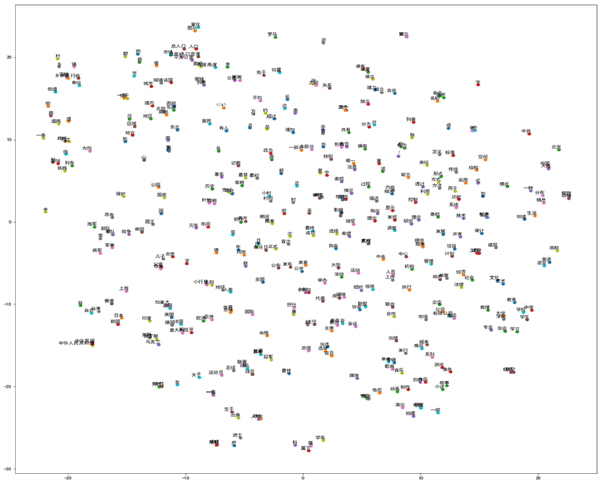``````!pip install gensim

frommodels importKeyedVectors

result1 = word_vectors.most_similar(positive=['女人', '国王'], negative=['男人'])

print("{}: {:.4f}".format(*result1))

print(word_vectors.doesnt_match("天才傻瓜 疯子 牛肉".split())) ``````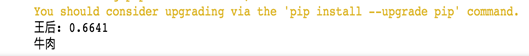``````!pip install wordcloud

importpyplot as plt

fromwordcloud import WordCloud

...

#输入一个词找出相似的前100个词

one_corpus = ["美丽"]

result = word_vectors.most_similar(one_corpus,topn=100)

#将返回的结果转换为字典,便于绘制词云

word_cloud = dict()

forsim inresult:

word_cloud[sim] = sim

#绘制词云

draw_word_cloud(word_cloud) ``````notebook实例C5 2xlarge \$0.538/小时，计费使用一小时；

GPU训练实例 P3 2xlarge \$4.284/小时，计费使用292秒；

S3 存储成本 \$0.023 每GB/月，存储大约3GB；

## 本篇作者